Showing 141 to 150 of 338 results.
0%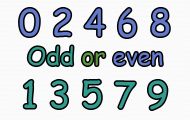## Odd or even numbers

• Even
• Odd
• Numbers
Here you get to practice recognizing even and odd numbers.
0%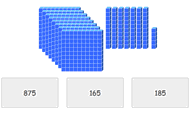## Place value with base ten blocks

• Counting
• Mental calculation
Look at the picture and count the hundreds. Then count the number of tens and after that the ones. Sum up and click on the correct number. Practice counting and improve your perception of numbers in t
0%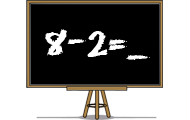## Subtraction

• Numbers
• Subtraction
• Mental calculation
Practice subtraction or minus in a skill training game. Find the difference between two numbers in different levels in this math game.
0%## Sums of two one-digit numbers

• 1-digit number
students will be adding one-digit numbers in this game.
0%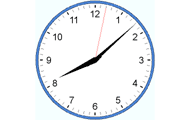## Tell time in English

• Clock
• Analog clock
• Numbers
Practice analog clock. Practice the basics such as full hours, half-hours, quarters and minutes. Watch the three clocks and choose the clock that match the requested time.
0%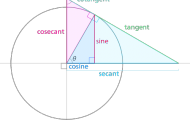## Trigonometry Vocabulary

• Trigonometry
• Functions
• Vocabulary
Practice the six trigonometric functions and other trigonometry definitions.
0%## Volume Concentration

• Volume
• Formula
• 3D figures
Match up the corresponding 3D figure to its volume formula.
0%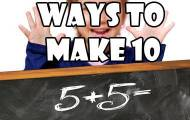## Ways to make 10

• Number bonds
• Make 10
These friends of ten activities really help students learn ways to make ten in a fun and hands on way.
0%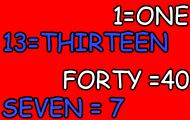## Writing numbers in words

• Numbers
• Digits
• Words
• Write
Practice how numbers are written in words. In English and both numbers to words and words to numbers.
0%## Planet order in the solar system

• Astronomy
• Planets
• Planet order
• Sun
Practice the order of the eight planets in the solar system.
Weekly top list
1.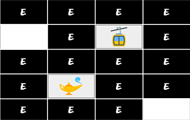Brain training
2.Brain training
3.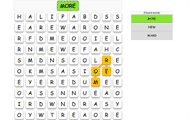English
4.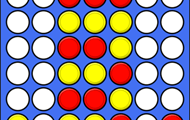Brain training
5.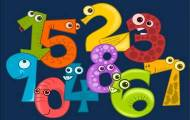Spanish

More plus membership benefits